JMSLTM Numerical Library 7.2.0
com.imsl.math

## Class SymEigen

• ```public class SymEigen
extends Object```
Computes the eigenvalues and eigenvectors of a real symmetric matrix. Orthogonal similarity transformations are used to reduce the matrix to an equivalent symmetric tridiagonal matrix. These transformations are accumulated. An implicit rational QR algorithm is used to compute the eigenvalues of this tridiagonal matrix. The eigenvectors are computed using the eigenvalues as perfect shifts, Parlett (1980, pages 169, 172). The reduction routine is based on the EISPACK routine `TRED2`. See Smith et al. (1976) for the EISPACK routines. Further details, some timing data, and credits are given in Hanson et al. (1990).

Let M = the number of eigenvalues,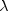= the array of eigenvalues, and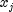is the associated eigenvector with jth eigenvalue.

Also, letbe the machine precision. The performance index,, is defined to be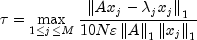While the exact value ofis highly machine dependent, the performance of `SymEigen` is considered excellent if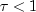, good if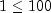, and poor if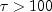. The performance index was first developed by the EISPACK project at Argonne National Laboratory; see Smith et al. (1976, pages 124-125).

Example
• ### Constructor Summary

Constructors
Constructor and Description
`SymEigen(double[][] a)`
Constructs the eigenvalues and the eigenvectors for a real symmetric matrix.
```SymEigen(double[][] a, boolean computeVectors)```
Constructs the eigenvalues and (optionally) the eigenvectors for a real symmetric matrix.
• ### Method Summary

Methods
Modifier and Type Method and Description
`double[]` `getValues()`
Returns the eigenvalues
`double[][]` `getVectors()`
Return the eigenvectors of a symmetric matrix of type `double`.
`double` `performanceIndex(double[][] a)`
Returns the performance index of a real symmetric eigensystem.
• ### Methods inherited from class java.lang.Object

`clone, equals, finalize, getClass, hashCode, notify, notifyAll, toString, wait, wait, wait`
• ### Constructor Detail

• #### SymEigen

`public SymEigen(double[][] a)`
Constructs the eigenvalues and the eigenvectors for a real symmetric matrix.
Parameters:
`a` - is the symmetric matrix whose eigensystem is to be constructed.
• #### SymEigen

```public SymEigen(double[][] a,
boolean computeVectors)```
Constructs the eigenvalues and (optionally) the eigenvectors for a real symmetric matrix.
Parameters:
`a` - a `double` symmetric matrix whose eigensystem is to be constructed
`computeVectors` - a `boolean`, true if the eigenvectors are to be computed
Throws:
`IllegalArgumentException` - is thrown when the lengths of the rows of the input matrix are not uniform.
• ### Method Detail

• #### getValues

`public double[] getValues()`
Returns the eigenvalues
Returns:
a `double` array containing the eigenvalues in descending order. If the algorithm fails to converge on an eigenvalue, that eigenvalue is set to NaN.
• #### getVectors

`public double[][] getVectors()`
Return the eigenvectors of a symmetric matrix of type `double`.
Returns:
a `double` array containing the eigenvectors. The j-th column of the eigenvector matrix corresponds to the j-th eigenvalue. The eigenvectors are normalized to have Euclidean length one. If the eigenvectors were not computed by the constructor, then null is returned.
• #### performanceIndex

`public double performanceIndex(double[][] a)`
Returns the performance index of a real symmetric eigensystem.
Parameters:
`a` - a `double` symmetric matrix
Returns:
a `double` scalar value indicating how well the algorithms which have computed the eigenvalue and eigenvector pairs have performed. A performance index less than 1 is considered excellent, 1 to 100 is good, while greater than 100 is considered poor.
Throws:
`IllegalArgumentException` - is thrown when the lengths of the rows of the input matrix are not uniform.
JMSLTM Numerical Library 7.2.0